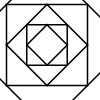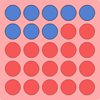#### You may also like### Tangram Tangle

If you split the square into these two pieces, it is possible to fit the pieces together again to make a new shape. How many new shapes can you make?### Shaping It

These pictures were made by starting with a square, finding the half-way point on each side and joining those points up. You could investigate your own starting shape.### All Change

There are three versions of this challenge. The idea is to change the colour of all the spots on the grid. Can you do it in fewer throws of the dice?

# Weighted Numbers

##### Age 5 to 7 Challenge Level:

Full Screen Version

Here is a balance. It has a hook on each end from which you can hang weights.

My weights are numbers - I have $1$s, $2$s, $3$s, $4$s, $5$s, $6$s, $7$s, $8$s, $9$s and $10$s.

If I put a $2$ on one side and two $1$s on the other side, it is balanced.
If I put a $3$ on one side, I would need three $1$s to make it balance. How else could I make it balance with just a $3$ on one side?

If I had a $3$ and a $4$ on one side and three $2$s on the other side, what do I need to add to make it balance and where?

I hang a $10$ on one side. How many different ways can you find of hanging numbers on the other side to make it balance?